Disclaimer: This is an example of a student written essay.

Any scientific information contained within this essay should not be treated as fact, this content is to be used for educational purposes only and may contain factual inaccuracies or be out of date.

# Investigation of the Relationship Between Spring Mass and Oscillation Time Using Hooke's Law

 ✅ Paper Type: Free Essay ✅ Subject: Physics ✅ Wordcount: 2682 words ✅ Published: 21st Sep 2021

Aim

This experiment is aimed to investigate the relationship between the mass that is being slotted at the end of spring and the time taken for the spring to make a 20 complete oscillations.

Hypothesis

As the mass of the end of the spring increases, the time period for the spring to complete 20 oscillation will also increase. This is because in newton’s second law which is F = ma, if the acceleration is being derived with the presence of time in its formula, it will be F = m(v-u)/t. Which proves that, mass is directly proportional to time. As the mass increases, the time will also increase.

Variables

Independent variable: Mass that is being slotted at the end of the spring (kg)

Dependent variable: Time period taken by the spring to make 20 complete oscillations (s)

Controlled variable:

• Spring constant
• Gravitational acceleration
• Length of spring
• Amount of spring oscillations
• Air resistance
• Amplitude of oscillations

Controlling the variables

Mass that is being slotted at the end of the spring:

The mass that is being slotted at the end of the spring is manipulated from 0.1kg, 0.2kg, 0.3kg, 0.4kg and 0.5kg.

Time period taken by the spring to make 20 complete oscillations:

With the aid of digital stopwatch, the time period is taken when the spring had completed in making 20 oscillations. This procedure is repeated 3 times and an average reading is taken.

Spring constant:

The spring constant is kept constant so that the results are relative to each other. It will be controlled by using the same spring throughout the whole experiment.

Gravitational acceleration:

The gravitational acceleration is kept constant by conducting the experiment in the same place until the end.

Amount of spring oscillations:

The amount of oscillations is controlled to 20 so that the results are more accurate. The exact results will be divided by 20 afterwards.

Air resistance:

Due to the place that the experiment is being conducted in a room, the fan and the air conditioner are switched off to reduce the air resistance. The experiment is conducted in the same place until the end to fix the air resistance.

Amplitude of oscillations:

The amplitude will be controlled each time the mass is increased by using the same length in extending the spring to make is oscillate. For each trial, the spring is extended 5cm downwards. This procedure will be aided by a meter ruler.

Materials list

1. Retort stand
2. Clamp and stand
3. Meter ruler
4. Mass holder
5. Slotted mass
6. Digital stopwatch
7. Spring

Diagram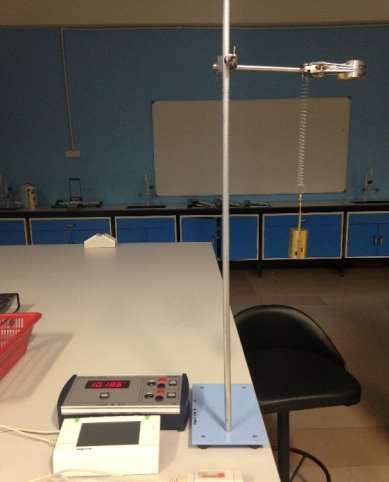Method

1. Set up the apparatus as shown in the diagram, with one end of the spring attached to the horizontal support on the clamp stand.
2. Attach the slotted mass of 0.1kg at the end of the spring.
3. Put the meter ruler next to spring and measure the extension.
4. Pull the slotted mass holder down to 5cm and then release it.
5. Let it oscillates for 20 times. Take the time taken for the spring to oscillate completely for 20 times using digital stopwatch.
6. Repeat step 4 to 6 two more times.
7. Record the data each time and take an average reading.
8. Repeat step 2 to 7 by using different slotted mass, from 0.2kg, 0.3kg, 0.4kg, and 0.5kg.

Results

 Mass that is being slotted (M) / kg (±0.01kg) Time taken for 20 oscillations / s (±0.01s) Average time taken for 20 oscillations / s (±0.01s) Time taken for one oscillation (T) / s (±0.01s) First try Second try Third try 0.10 4.52 4.13 4.62 4.42 0.22 0.20 6.31 6.50 5.92 6.24 0.31 0.30 8.4 8.5 8.8 8.6 0.43 0.40 10.2 10.5 9.8 10.2 0.51 0.50 11.4 10.7 11.8 11.3 0.57

Data presentation

A graph to show the relationship between the mass that is being slotted at the end of the spring, M, and the time taken for one oscillation, T.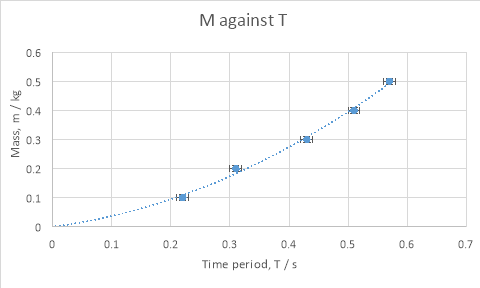Data analysis

The relationship between mass that is being slotted at the end of the spring and the time taken for spring to oscillate one cycle appears to be non-linear. The data therefore will be processed in order to find a relationship between this two variables either it is directly proportional or not. The graph is parabolic. Hence the T can be manipulated to become T2 so that the graph can be plotted with a variable of mass against T2.

Data processing

Table 2 – Mass that is being slotted at the end of spring, M, and squared of time taken for one oscillation, T2

 Mass being slotted (M) / kg (±0.01kg) Time taken for one oscillation (T) / s (±0.01s) Time taken for one oscillation squared (T2) / s (±0.02s) 0.10 0.22 ± 4.5% 0.04 ± 0.36 x 10-2 0.20 0.31 ± 3.2% 0.10 ± 0.64 x 10-2 0.30 0.43 ± 2.3% 0.18 ± 0.83 x 10-2 0.40 0.51 ± 2.0% 0.26 ± 1.04 x 10-2 0.50 0.57 ± 1.8% 0.32 ± 1.15 x 10-2

Presentation and analysis of the processed data

A graph shows the relationship between the mass that is being slotted at the end of the spring, M, and the squared of time taken for one oscillation,T2.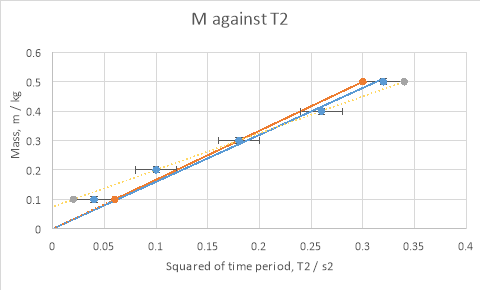Analysis of the graph

Gradient of best fit line = 0.50/0.32 = 1.56 kgs-2

Gradient of steepest line = 0.50/0.30 = 1.67 kgs-2

Gradient of shallowest line = 0.42/0.34 = 1.24 kgs-2

For the second graph, it was proved that mass that is being slotted at the end of spring is direclty proportional to the squared of time period.

Mathematically, m α T2

After the investigation and the experiment that had been done, it was found that the formula relating the mass that is being slotted at the end of spring and time period of an oscillating spring is :

We know that ; T = 2π/Æœ

Where Æœ = k(constant) in this investigation.

Therefore,

T = 2π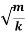T2 =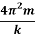Which is of the form,

y = mx + c (equation of straight line)

From the investigation, y is m, m is 4π2/k and x is T2.

The gradient of the line is therefore equal to 2/k , we can now find the spring constant:

Therefore, k on the best fit line ;

1.56 = 1/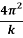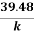= 1/1.56

k = 61.59 Nm-1

The range of uncertainty in this value can be calculated using both the maximum and the minimum lines on the graph.

1.67 = 1/= 1/1.67

k = 65.93 Nm-1

1.24 = 1/= 1/1.24

k = 48.96 Nm-1

Therefore the spring constant, k is in the range of 48.96 Nm-1 to 65.59 Nm-1.

Conclusion

The aim of this experiment is to investigate the relationship between the mass that is being slotted at the end of spring and time period of oscillation. As the hypothesis being made earlier that mass would be directly proportional to time period of oscillation, it is clearly was wrong as the graph of mass against time period is obviously non-linear. The second graph of mass against squared of time period is however turned out to be linear and therefore it can be concluded that mass is directly proportional to the squared of time period.

After the investigation, this conclusion is supported as the equation for time period of an oscillating spring is

T = 2πT2 =So, T2 α m

The gradient of straight line was then used to calculate the spring constant, k, for the spring used in this experiment. This is because the gradient is equal to 1/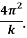The value can be compared to the theoretical value by using Hooke’s so as to verify the result whether it is plausible or not. When the spring is acted a force of 1N, the extension was seen to be 1.6cm. The spring constant can be determine by using the formula of Hooke’s law;

F = kx

k = 1/0.016

k = 62.5 Nm-1

Calculating percentage deviation: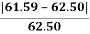x 100% = 1.5%

The actual value and the theoretical value is not that far and only 1.5% in the percentage deviation. In conclusion, it can be said that this experiment is successful and the results are accurate.

Evaluation

The method and apparatus used worked well throughout the whole experiment. The results obtained are differ from the actual results. This is because they are maybe some mistakes were made during taking the reading or making the experiment works. There are some improvements that were made when collecting the data that were not stated in the original plan.

1. Parallax error occurs when reading the ruler which the recorder’s eye level is not perfectly perpendicular to the ruler.
2. The slotted mass were considered to be the same. Just one of the slotted mass was weighted and for one slotted mass the mass is 0.1kg. Without hesitation, the other slotted mass were all considered to be 0.1kg in mass too. This may have produced a systematic error, depending on how accurate the masses were and consistency of their inaccuracy.
3. When lighter slotted mass were used, the oscillations was so fast. Random errors can occur. Suggested that, the spring should be let to oscillation more so that the results will be more precise.

Suggested improvements

The investigation could has been more accurate and precise if the following modifications were to be taken:

1. Make sure that the eye is perpendicular to the ruler when taking the reading when doing the extension of spring. This will avoid parallax error.
2. Use another ruler to point at the ruler when taking the reading. This will aid to read the meter ruler easier and more precise. This will avoid parallax error.
3. To make the time taken more accurate, use ultra-sonic motion detector that is placed below the oscillating spring. The ultra-sonic motion detector will collect data more precisely because it does not involve the human interaction which is affected by human reaction.
4. Take more reading and take the average as the lesser the reading are taken, it will create more random errors.

View all

## DMCA / Removal Request

If you are the original writer of this essay and no longer wish to have your work published on UKEssays.com then please:

Related Services

Prices from

BHD57

Approximate costs for:

• 1000 words
• 7 day deliveryHumanity University

Dedicated to your worth and value as a human being!

Related Lectures